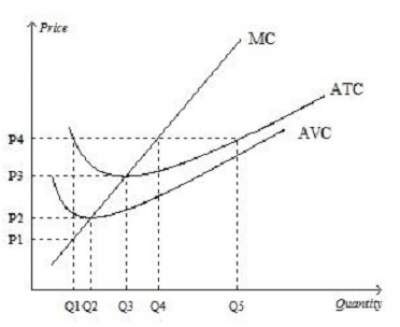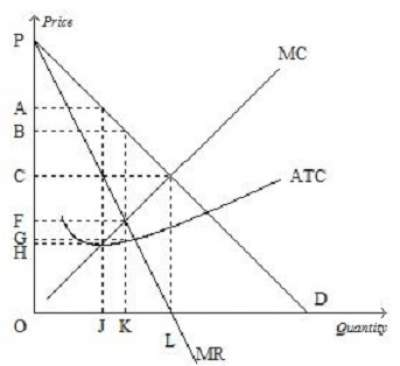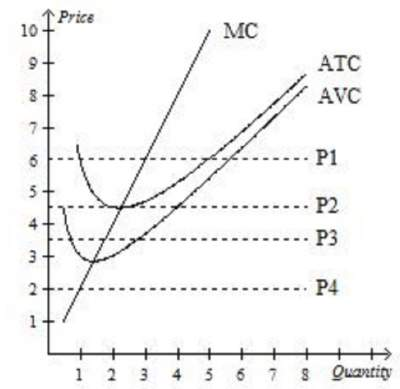# Overall Practice Test-1 on Economics: Solutions

Question 1: Economics deals primarily with the concept of ..........
1. Money.
2. Scarcity.
3. Power.
4. Banking.
5. none of the above

Question 2: Which of the following statements is correct? .....
1. A competitive firm is a price maker, whereas a monopolist is a price taker.
2. Both a competitive firm and a monopolist are price makers.
3. A competitive firm is a price taker, whereas a monopolist is a price maker.
4. Both a competitive firm and a monopolist are price takers.
5. 1 and 2

Question 3: In a market economy, supply and demand determine.............
1. both the quantity of each good produced and the price at which it is sold
2. the price at which each good is sold, but not the quantity of each good produced.
3. neither the quantity of each good produced nor the price at which it is sold.
4. the quantity of each good produced, but not the price at which it is sold.
5. 1 and 4

Question 4: Which of the following is not a characteristic of a monopoly? ......
2. barriers to entry.
3. one seller.
4. a product without close substitutes.
5. 2 and 3

Question 5: When quantity demanded decreases at every possible price, we know that the demand curve has ......
1. shifted to the right.
2. shifted to the left.
3. not shifted; rather, we have moved along the demand curve to a new point on the same curve.
4. none of the above.

Question 6: The opportunity cost of going to college is .........
1. the value of the best opportunity a student gives up to attend college.
2. clothing, books, meals, transportation, tuition, lodging, and any other expenses.
3. zero for students who are fortunate enough to have all of their college expenses paid by someone else.
4. zero, since a college education will allow a student to earn a larger income after graduation.

Question 7: Which of the following statements is correct?
1. Sellers determine both demand and supply.
2. Buyers determine both demand and supply.
3. Buyers determine demand and sellers determine supply.
4. Buyers determine supply and sellers determine demand.

Question 8: Explicit costs
1. include income that is forgone by the firm's owners.
2. require an outlay of money by the firm.
3. include all of the firm's opportunity costs.
4. all of the above.

Question 9: Amy decides to open her own business and earns \$75,000 in accounting profit the first year.
When deciding to open her own business, she turned down two separate job offers with annual salaries of \$40,000, \$50,000, and \$60,000.
What is Amy's economic profit from running her own business?
1. \$35,000
2. \$20,000
3. −\$15,000
4. \$15,000

Assume that the table below shows the expenses of a local IT startup ABC.
Q TC FC VC MC AFC AVC ATC
0 \$150 \$150 \$0 .... .... .... ....
1 \$250 A B C D E F
2 G H I \$220 J K L
3 M N O P Q \$220 R

Question 10: Find A.
1. \$150
2. \$100
3. \$75
4. \$45

Question 11: Using the same table of the company ABC, find G.
1. \$255
2. \$265
3. \$470
4. \$275
Explanation: MC = ΔT / ΔQ = \$220
This implies that T2 − T1 = \$220 and since T1 = \$250 then T2 = \$220 + \$250 = \$470

Question 12: Based on the graph below when price rises from P3 to P4, the firm finds that........1. fixed costs decrease as output increases from Q3 to Q4.
2. it can earn a positive profit by increasing production to Q4.
3. profit is still maximized at a production level of Q3
4. average revenue exceeds marginal revenue at a production level of Q4

Question 13: Using the same figure in question 12, firms would be encouraged to enter this market for all prices that exceed .....
1. P2
2. P3
3. P4
4. P5
5. none of the above

Question 14: The fundamental source of monopoly power is ............
1. profit.
2. barriers to entry.
3. decreasing average total cost.
4. a product without close substitutes.

Question 15: See the graph below and find the mathematical formula to calculate the monopolist's profit.1. (B − C) × J
2. (A − G) × L
3. (B − C) × K
4. (B − G) × K
5. none of the above

Question 16: A profit-maximizing monopoly's will produce an output equal to .......1. Q3
2. Q1
3. Q2
4. Q4

Question 17: Which of the following pairs illustrates the two extreme examples of market structures?
1. competition and monopoly
2. competition and oligopoly
3. monopoly and monopolistic competition
4. oligopoly and monopolistic competition

Question 18: A market structure with only a few sellers, each offering similar or identical products, is known as .....

1. monopoly
2. perfect competition
3. oligopoly
4. monopolistic competition

Question 19: A distinguishing feature of an oligopolistic industry is the tension between .....

1. short-run decisions and long-run decisions.
2. producing a small amount of output and charging a price above marginal cost.
3. profit maximization and cost minimization.
4. cooperation and self interest.

Question 20: Goods with many close substitutes tend to have .....

1. more elastic demands.
2. income elasticities of demand that are negative.
3. price elasticities of demand that are unit elastic.
4. less elastic demands.

Question 21: The adage, "There is no such thing as a free lunch," means .....

1. send people gifts during their annual anniversary.
3. the cost of living is always decreasing.
4. Some costs are not included in the price of a product.

Question 22: The law of demand states that, other things equal,

1. when the price of a good falls, the quantity demanded of the good rises.
2. when the price of a good rises, the demand for the good falls.
3. when the price of a good rises, the quantity demanded of the good rises.
4. when the price of a good falls, the demand for the good rises.

Question 23: Rational people make decisions at the margin by

2. having a tough attitute.
3. comparing marginal costs and marginal benefits.
4. be politically correct.

Question 24: A surplus exists in a market if ......

1. there is an excess demand for the good.
2. the situation is such that the law of supply and demand would predict an increase in the price of the good from its current level.
3. the current price is above its equilibrium price.
4. quantity demanded exceeds quantity supplied.
Question 25: Clark rents 3 movies per month when the price is \$2.00 per rental and 5 movies per month when the price is \$1.50 per rental.
Clark'S demand demonstrates the law of ......

1. income.
2. demand.
3. supply.
4. price.

Question 26: A typical society strives to get the most it can from its scarce resources.
At the same time, the society attempts to distribute the benefits of those resources to the members of the society in a fair manner.
In other ...

1. efficiency and equality.
2. guns and butter.
3. inflation and unemployment.
4. work and leisure.

Question 27: A rightward shift of a supply curve is called .....

1. a decrease in supply.
2. an increase in supply.
3. an increase in quantity supplied.
4. a decrease in quantity supplied.

Question 28: As new firms enter a monopolistically competitive market, profits of exisiting firms .....

1. rise, and product diversity in the market increases.
2. rise, and product diversity in the market decreased.
3. decline, and product diversity in the market decreases.
4. decline, and product diversity in the market increases.

Question 29: law of supply states that, other things equal,

1. an increase in price causes quantity supplied to increase.
2. an increase in price causes quantity supplied to decrease.
3. an increase in quantity supplied causes price to increase.
4. an increase in quantity supplied causes price to decrease.

Question 30: At the equilibrium price, the quantity of the good that buyers are willing and able to
buy exactly equals the quantity that sellers are willing and able to sell.

1. is greater than the quantity that sellers are willing and able to sell.
2. is less than the quantity that sellers are willing and able to sell.
3. exactly equals the quantity that sellers are willing and able to sell.
4. all of the above.

Question 31: Economists assume that the typical person who starts his own business does so with the intention of....

1. become a leader in his country.
2. obtaining the highest number of "Likes" in his industry.
3. maximizing profits.
4. minimizing costs.

Question 32: Elasticity is ....

1. the study of how the allocation of resources affects economic well-being.
2. a measure of how much buyers and sellers respond to changes in market conditions.
3. the maximum number of buyers who will pay for a good.
4. the maximum number of sellers who will sell the good.
5. the value of everything a seller must give up to produce a good.

Question 33: The price elasticity of demand measures how much ....

1. quantity demanded responds to a change in price.
2. demand responds to a change in supply.
3. price responds to a change in demand.
4. quantity demanded responds to a change in income.

Question 34: ABC company produced 500 units of output but sold only 450 of the units it produced.
The average cost of production for each unit of output produced was \$150.
Each of the 450 units sold was sold for a price of \$130. Total profit for the ABC company would be ....

1. −\$13,500.
2. −\$15,500.
3. −\$14,500
4. −\$16,500
Explanation:
TC = 500 Ļ \$150 = \$75,000; Revenues = 450 × \$130 = \$58,500 and hence Total Profit = \$58,500 − \$75,000 = −\$16,500

Question 35:Which of the following statements is correct? ...

1. Opportunity costs equal total minus implicit costs.
2. Economists consider opportunity costs to be included in a firm's costs of production.
3. Economists consider opportunity costs to be included in a firm's total profits.
4. None of the above